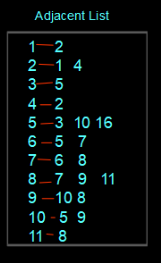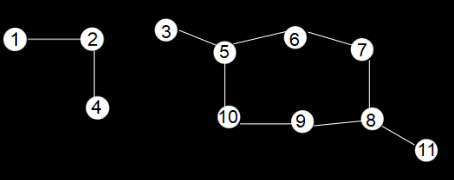# Detect a cycle in Undirected Graph : Breadth-First Search

Problem Statement: Given an undirected Graph, check for a cycle using BFS (Breadth-First Search) Traversal.

```Example:

Input:Output: Yes```
```Explanation:Since 8 is a point where loop is formed```

### Solution

Disclaimer: Don’t jump directly to the solution, try it out yourself first.

Solution 1:

Intuition: The intuition behind this is to check for the visited element if it is found again, this means the cycle is present in the given undirected graph.

for(i= 1->N)

{

if(!vis[i])

if(cyclebfs(i)) // if cycle is found , we return true

Return True;

}Approach

For the first component, firstly we insert the first element of the graph by marking it as visited in the Queue having prev of the first element as -1. Now we pop out that element and traverse the adjacent element list for that and then insert the elements in the Queue and continue the process until no adjacent element is found for the present element. Here, we can see that no loop is formed. So, we return false for the first component.For the second component, a similar process will be done as we have done for the first component. Here we will be able to see that when we come to 7, 8 is one of the next adjacent ones which matters to us, and also, it is already marked as positive. This means someone is linked already with this element. So, it forms a loop and we return true finally.Code:

## C++ Code

``````#include <bits/stdc++.h>
using namespace std;
class Solution
{
public:
bool checkForCycle(int s, int V, vector<int> adj[], vector<int> &visited)
{
// Create a queue for BFS
queue<pair<int, int>> q;
visited[s] = true;
q.push({s, -1});
while (!q.empty())
{
int node = q.front().first;
int par = q.front().second;
q.pop();

{
if (!visited[it])
{
visited[it] = true;
q.push({it, node});
}
else if (par != it)
return true;
}
}
return false;
}
{
vector<int> vis(V, 0);
for (int i = 0; i < V; i++)
{
if (!vis[i])
{
return true;
}
}
}
};

{
}
int main()
{

Solution obj;
if(num==1)
cout<<"Yes"<<endl;
else
cout<<"No"<<endl;

return 0;
}``````

Output:

Yes

Time Complexity: O(N+E),  N is the time taken and E is for traveling through adjacent nodes overall.

Space Complexity: O(N+E) +  O(N) + O(N) , space for adjacent list , array and queue

## Java Code

``````import java.util.*;
import java.lang.*;
import java.io.*;
class GFG
{
public static void main(String[] args) throws IOException
{

int V = 5;
for(int i = 0; i < V; i++)

Solution obj = new Solution();
if(ans)
System.out.println("Yes");
else
System.out.println("No");
}
}

class Node {
int first;
int second;
public Node(int first, int second) {
this.first = first;
this.second = second;
}
}
class Solution
{
static boolean checkForCycle(ArrayList<ArrayList<Integer>> adj, int s,
boolean vis[])
{
Queue<Node> q =  new LinkedList<>(); //BFS
vis[s] =true;

while(!q.isEmpty())
{
int node = q.peek().first;
int par = q.peek().second;
q.remove();

{
if(vis[it]==false)
{
vis[it] = true;
}

else if(par != it) return true;
}
}

return false;
}
public boolean isCycle(int V, ArrayList<ArrayList<Integer>> adj)
{
boolean vis[] = new boolean[V];
Arrays.fill(vis,false);
for(int i=0;i<V;i++)
if(vis[i]==false)
return true;

return false;
}
}``````

Output:

Yes

Time Complexity: O(N+E),  N is the time taken and E is for traveling through adjacent nodes overall.

Space Complexity: O(N+E) +  O(N) + O(N) , space for adjacent list , array and queue